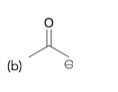Problem: Draw the conjugate acid for each of the following bases

FREE Expert Solution
94% (132 ratings)
Problem Details

Draw the conjugate acid for each of the following basesWhat scientific concept do you need to know in order to solve this problem?

Our tutors have indicated that to solve this problem you will need to apply the Acid and Base Conjugates concept. If you need more Acid and Base Conjugates practice, you can also practice Acid and Base Conjugates practice problems .

What is the difficulty of this problem?

Our tutors rated the difficulty of Draw the conjugate acid for each of the following bases... as low difficulty.

How long does this problem take to solve?

Our expert Organic tutor, Jonathan took 2 minutes to solve this problem. You can follow their steps in the video explanation above.

What professor is this problem relevant for?

Based on our data, we think this problem is relevant for Professor Miessein's class at HUNTER.

What textbook is this problem found in?

Our data indicates that this problem or a close variation was asked in . You can also practice practice problems .